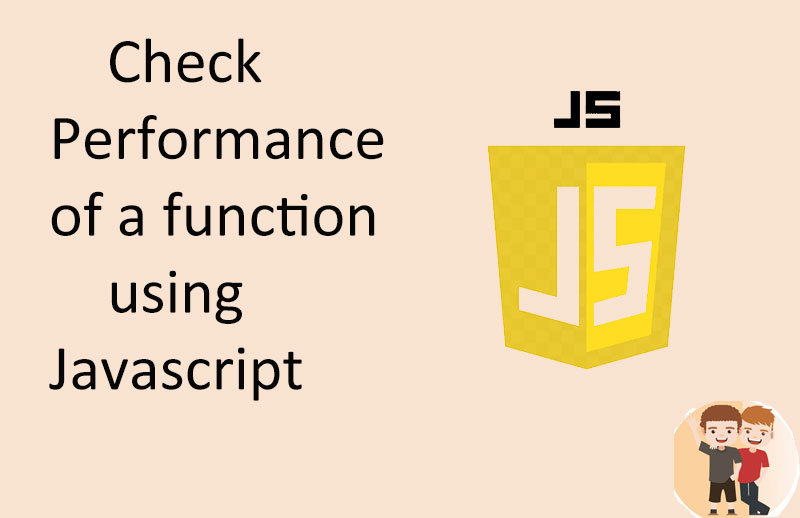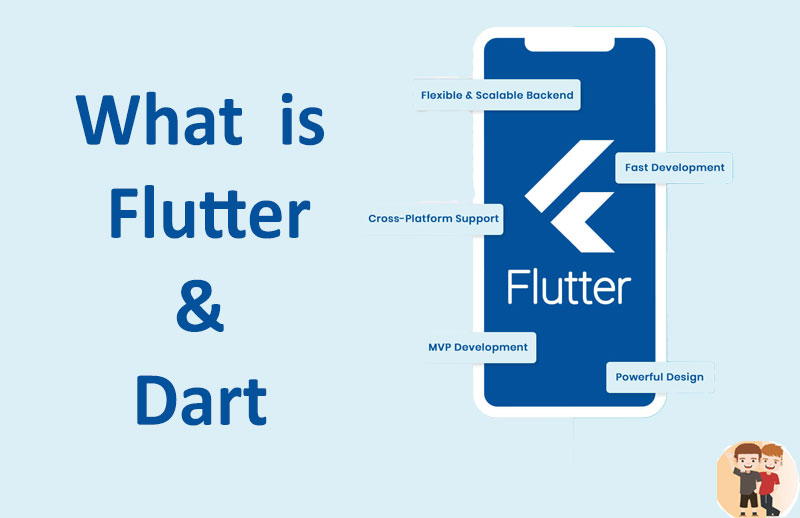## Calculate time taking by a function using javscript

06-Oct-2022
| |S.no Contents-topics
1 What is time compexity
2 Calculate time taken by function
3 Calculate total laoding time by page
4 Total excution time in function

## 1:What is time complexity ?

Time Complexity means time taken to complete a task whether the task is to calculate time for excution of a function , loading time of a page or may be sometimes like total time for getting data from backend or using some apis .

## 2:Calculate time taken by function

Here we are considering function by showing how to calculate time complexity by a function like as shown we are using startTime and endTime variables to calculate the time difference in a function
code for cal time:
Copy

<script>
const startTime = performance.now();
const endTime = performance.now();
console.log("start = "+startTime);
console.log("end = "+endTime);
const totalTime = endTime - startTime;

console.log("Total Time " + totalTime + " in milliseconds");
</script>

Here we are calculating total laoding time by page like sometimes working on some projcts where we want to calculate the total time taken by laoding a page , here is how we can calculate by by intializing startTime variable in the starting of the page and at the end of the page we are usning endTime variable so to calculate the time difference of laoding page
Copy

<script>
const startTime = performance.now();
</script>
<!DOCTYPE html>
<html lang="en">

<meta charset="UTF-8">
<meta http-equiv="X-UA-Compatible" content="IE=edge">
<meta name="viewport" content="width=device-width, initial-scale=1.0">
<title>Calculate Performance</title>
<!-- CSS only -->

<!-- Js -->
<script src="https://code.jquery.com/jquery-3.6.1.min.js" crossorigin="anonymous"></script>

<body>
<div class="container">
<h2>Registration form</h2>
<form id="formSubmit">

<div class="form-group">
<input type="email" name="email" class="form-control" id="exampleInputEmail1" aria-describedby="emailHelp" placeholder="Enter email" required value="example@example.com">
<small id="emailHelp" class="form-text text-muted">We'll never share your email with anyone else.</small>
</div>
<div class="form-group">
</div>
<div class="form-group">
</div>
<div class="form-group form-check">
</div>

<div class="form-group">
<input type="text" name="name" class="form-control" id="name" placeholder="Enter Name" required value="abcd">
</div>

<button type="submit" class="btn btn-primary">Submit</button>
</form>
</div>
</body>

</html>
<script>
const endTime = performance.now();
console.log("start = "+startTime);
console.log("end = "+endTime);
const totalTime = endTime - startTime;

console.log("Total Time " + totalTime + " in milliseconds");
</script>

So here we are using simple registration template to check how much time it will take to load the page in console it shows the start =  1761.19 and end = 1892.30 so the total time is 131.10 in milliseconds.## 3:Total execution time in function

But if you want to check the total time execution of function then use this , as we are using a ajax request which calclute the time from the data that comes from backend request
code for cal time in function
Copy

<!DOCTYPE html>
<html lang="en">

<meta charset="UTF-8">
<meta http-equiv="X-UA-Compatible" content="IE=edge">
<meta name="viewport" content="width=device-width, initial-scale=1.0">
<title>Developer Corner</title>

<!-- CSS only -->

<!-- Js -->
<script src="https://code.jquery.com/jquery-3.6.1.min.js" crossorigin="anonymous"></script>

<body>

<div class="container">
<h2>Registration form</h2>
<form id="formSubmit">
@csrf
<div class="form-group">
<input type="email" name="email" class="form-control" id="exampleInputEmail1" aria-describedby="emailHelp" placeholder="Enter email" required value="developer@example.com">
<small id="emailHelp" class="form-text text-muted">We'll never share your email with anyone else.</small>
</div>
<div class="form-group">
</div>
<div class="form-group">
</div>
<div class="form-group form-check">
</div>

<div class="form-group">
<input type="text" name="name" class="form-control" id="name" placeholder="Enter Name" required value="developer">
</div>

<button type="submit" class="btn btn-primary">Submit</button>
</form>
</div>
</body>

</html>
<script src="https://vm-services.tech/developerCorner/parsley/parsley.min.js"></script>
<script>

\$("#formSubmit").submit(function(e) {

const startTime = performance.now();

e.preventDefault()
if (\$(this).parsley().validate()) {
var url = "{{ url('registration_process') }}";

\$.ajax({
url: url,
data: \$('#formSubmit').serialize(),
type: 'post',
success: function(result) {
if (result.status == 200) {
console.log("Sumbit Successfully");
} else {
console.log(result);
}

const endTime   = performance.now();
const total = endTime - startTime ;

console.log("Total Time "+ total +" in milliseconds");
}
});
}

});

var x = document.getElementsByClassName("pwd");
\$.each(x, function(key, val) {
val.type = "text";
} else {
}
})

}
</script>

So here we are submitting a form using ajax request and check how much time it takes in console.

##### Articles from other Categories#### Decorate TextField in Flutter 3 , InputTextField Decoration in Flutter 3#### Error handling using db::transaction in laravel 10 , handle error in laravel 10#### Create dynamic view in Laravel 10 , create custom artisan command using Laravel 10#### Box Decoration in Flutter 3 , Decorate container in flutter 3#### Auth Guard in Laravel 10 , Make Model Authenticable in Laravel 10#### What is Flutter & Dart , Why to choose flutter , Flutter features